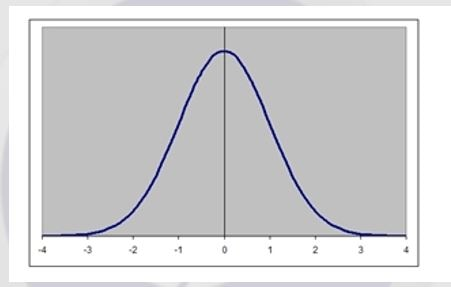# Six Sigma Statistics

Throughout a Six Sigma project, it is evident that there is a large amount of reliance upon statistical analysis.  In the very early stages, we stated that its use of statistics is one of the key elements that separate Six Sigma from many other quality methodologies.  They enable informed analysis of data from sampling through to modeling potentials.  They help the practitioner make an informed decision with validity as the project progresses.The main elements of statistics used within Six Sigma are covered in the following section.

Basic Statistics:
This includes understanding the core principles and mathematics at the heart of statistics – how to work out averages and variances among other commonly used terms. These can be seen below:

Average – there are 3 types of averages most commonly used in statistics: the mode, median, and mean average.
The mode or modal point is a range that occurs most frequently. So, if you have a set of results, the mode can be attributed to that result found the most often.

The median average is the center point of a range of values, so if you have values ranging from 10 to 50, the median point will occur at 30.

The mean average is the one most frequently used and referred to as average, but it requires math to work it out.
It is worked out by adding together all of the values and dividing by the number of total values. For example, if you receive 50 responses to an item and the results are all added together, then this result is divided by 50 to give the mean average. The mean average will often be represented by a (“x bar”).

Variance is a calculation across a range of data that shows how spaced out the information is.  Similarly the standard deviation will be a spacing value of information.  The variance is the result of squaring the standard deviation.  So, where the Standard Deviation (SD) is often represented by σ (the Greek letter sigma), then variance (VAR) will be represented by σ 2 (sigma squared).

The standard deviation is used frequently in determining confidence levels and probability of a result falling within a certain range.   But, you can see where the Six Sigma comes from, where SD relates to a sigma, and that statistics states that you can be 99.99966% confident of a containment within 3 sigmas on either side of the mean average.

There are many other statistical formulas, but these are the core groundwork for any and all statistics work. If you can understand these, then you can understand most.  The use of graphical representation is fundamental within statistics. There are many common types of graphs, none more so than the normal distribution graph.
This graph is shaped like a bell and often referred to as a bell chart. An example can be seen below. In a normal distribution curve, the mean, median, and mode are all the same value.

Charts and graphs allow us to plot occurrences, frequencies, occasions, and all manner of data together to be viewed without the need for the numbers.  They are often used to see patterns or shapes to try and make formulas fit into some shape of equation. There are some statistics around the line of best fit, and that relates to a frequency line showing the line of most likely results, almost like a rolling average of results based on the moving data displayed. An example of this can also be seen below.